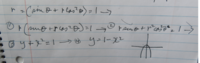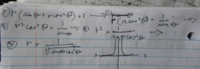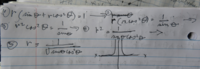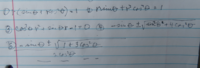# polar to rectangular

#### allegansveritatem

##### Full Member
The instruction is to find an equation in x and y that has the same graph as the given polar equation. The given equation is:I rendered this in to the language of x and y thus:Also, because of the way my calculator wants polar equations to be input into the graphing facility rendered the given polar equation into a form where there is only one r in the equation and it is singular with no exponents and positive as follows:So...what did I do here that got me two such different graphs from expressions I thought were equal?

#### skeeter

##### Elite Member
[MATH]r = \frac{-\sin{t} \pm\sqrt{1+3\cos^2{t}}}{2\cos^2{t}}[/MATH]
either plus or minus the radical matches your parabola, except at [MATH]t = \frac{\pi}{2}, \frac{3\pi}{2}[/MATH]

Last edited:

#### lex

##### Full Member
So...what did I do here that got me two such different graphs from expressions I thought were equal?You went from [MATH]r\sin\theta + r^2\cos^2\theta =1 \hspace1ex \text{ to } \hspace1ex r^2\cos^2\theta =\frac{1}{\sin\theta}[/MATH]It would be [MATH]r^2\cos^2\theta =1 - r\sin\theta[/MATH](which isn't much use anyway).

I infer that skeeter solved the quadratic, for r:
[MATH]r^2\cos^2\theta+ r\sin\theta -1=0[/MATH]

#### allegansveritatem

##### Full Member
[MATH]r = \frac{-\sin{t} \pm\sqrt{1+3\cos^2{t}}}{2\cos^2{t}}[/MATH]
either plus or minus the radical matches your parabola, except at [MATH]t = \frac{\pi}{2}, \frac{3\pi}{2}[/MATH]
I will have to copy this and work on figuring out what you did. I shall return with results. Thanks

#### allegansveritatem

##### Full Member
View attachment 27400

You went from [MATH]r\sin\theta + r^2\cos^2\theta =1 \hspace1ex \text{ to } \hspace1ex r^2\cos^2\theta =\frac{1}{\sin\theta}[/MATH]It would be [MATH]r^2\cos^2\theta =1 - r\sin\theta[/MATH](which isn't much use anyway).

I infer that skeeter solved the quadratic, for r:
[MATH]r^2\cos^2\theta+ r\sin\theta -1=0[/MATH]
yes, I see that now...thanks

#### lex

##### Full Member
yes, I see that now...thanks
Good.
(Interesting name - 'allegans veritatem' - the only reference I can see is to: Animadvertendum, quod allegans veritatem aliter haberi non posse id debet probare - a good principle for a mathematical site. Is this the source of the name?)

Last edited:

#### jonah2.0

##### Full Member
Beer soaked ramblings follow.
Given how Desmos almost always graphs any implicit equation, I was quite surprised that it wasn't able to graph r(sintheta+rcos^2theta)=1 as given and must be rewritten as skeeter did in order to graph it.

•nasi112

#### allegansveritatem

##### Full Member
View attachment 27400

You went from [MATH]r\sin\theta + r^2\cos^2\theta =1 \hspace1ex \text{ to } \hspace1ex r^2\cos^2\theta =\frac{1}{\sin\theta}[/MATH]It would be [MATH]r^2\cos^2\theta =1 - r\sin\theta[/MATH](which isn't much use anyway).

I infer that skeeter solved the quadratic, for r:
[MATH]r^2\cos^2\theta+ r\sin\theta -1=0[/MATH]
so...I spent a while puzzling over just what you did to get this equation. It didn't occur to me that is was in the form of the quadratic formula but I recalled that the post that comes after yours from Skeeter said something about your solving for something so I went back and reread his post and the light went on: Oh! He made a quadratic equation and solved for r! With that in mind I did this:It works! Thanks again.

#### allegansveritatem

##### Full Member
Good.
(Interesting name - 'allegans veritatem' - the only reference I can see is to: Animadvertendum, quod allegans veritatem aliter haberi non posse id debet probare - a good principle for a mathematical site. Is this the source of the name?)
No, I think the line you quote here has something to do with an old moral tract. Mine partially comes from a tag: allegans contraria non est audiendus which means something like: He who contradicts himself ought not to be listened to (or, is not to be allowed to testify). So I took the gerund, audiendus, and mated it with the accusative case of veritas to suggest that I am telling the truth--to the best of my inconsiderable ability.

Last edited:

#### allegansveritatem

##### Full Member
Beer soaked ramblings follow.
Given how Desmos almost always graphs any implicit equation, I was quite surprised that it wasn't able to graph r(sintheta+rcos^2theta)=1 as given and must be rewritten as skeeter did in order to graph it.
I got my graphs for TI Nspire calculator. I also have HP Prime which can make a graph of almost any equation, except apparently when it comes to porlar quations, in whcih case you need the form r= etc

#### allegansveritatem

##### Full Member
[MATH]r = \frac{-\sin{t} \pm\sqrt{1+3\cos^2{t}}}{2\cos^2{t}}[/MATH]
either plus or minus the radical matches your parabola, except at [MATH]t = \frac{\pi}{2}, \frac{3\pi}{2}[/MATH]
Excuse me for not giving you the credit for the tip you gave me--I mixed your post up with Lex's. I again upload my results: so...I spent a while puzzling over just what you did to get this equation. It didn't occur to me that is was in the form of the quadratic formula but I recalled that the post that comes after yours from Lex said something about your solving for something so I went back and reread his post and the light went on: Oh! He made a quadratic equation and solved for r!•lex

#### lex

##### Full Member
No, I think the line you quote here has something to do with an old moral tract. Mine partially comes from a tag: allegans contraria non est audiendus which means something like: He who contradicts himself ought not to be listened to (or, is not to be allowed to testify). So I took the gerund, audiendus, and mated it with the accusative case of veritas to suggest that I am telling the truth--to the best of my inconsiderable ability.

allegans contraria non est audiendus - another legal principle, then.
allegans veritatem - a noble motto. I'm sure you are more than able to fulfil it.

#### allegansveritatem

##### Full Member
allegans contraria non est audiendus - another legal principle, then.
allegans veritatem - a noble motto. I'm sure you are more than able to fulfil it.
well...not always. But I work on it. A work in progress.